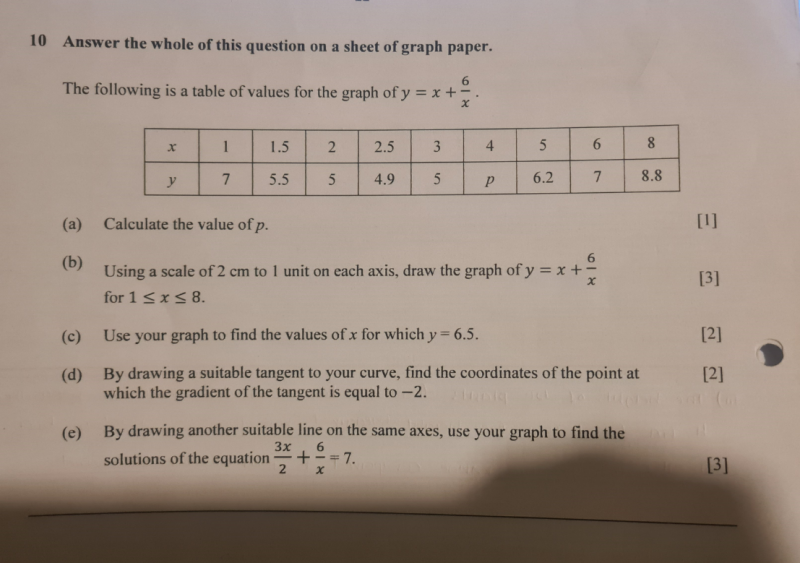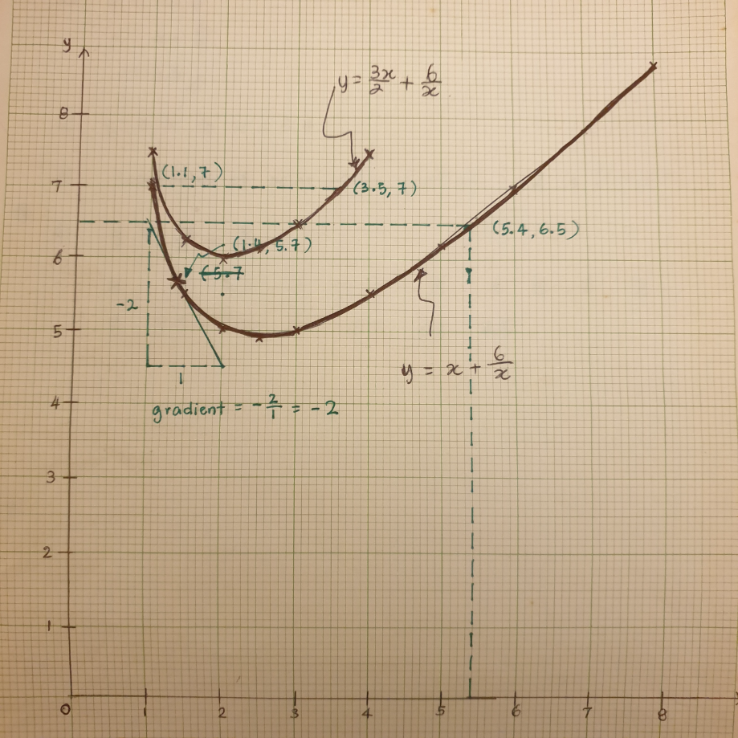# QuestionHello, can anyone help with these questions?

Thank you(a) y  = x + 6/x

p =  4 + 6/4   =5.5

(c)  y = 6.5  => x = 5.4

(d)  coordinates of point where gradient = -2 is (1.4, 5.7)

(e) y = 3x/2 + 6/x

plot this graph to obtain the solutions for  3x/2 + 6/x = 7

x         1              1.5              2             2.5              3            4

y        7.5           6.25            6            6.15          6.5          7.5

You can stop as long as the 2 end points are > 7 which is the solution you want to find on the graph.

So, from the graph, correct to 1 decimal place, the 2 possible solutions for x is 1.1 and 3.5

Note:   You need a curve rule to draw the curve in order to get a more accurate answer